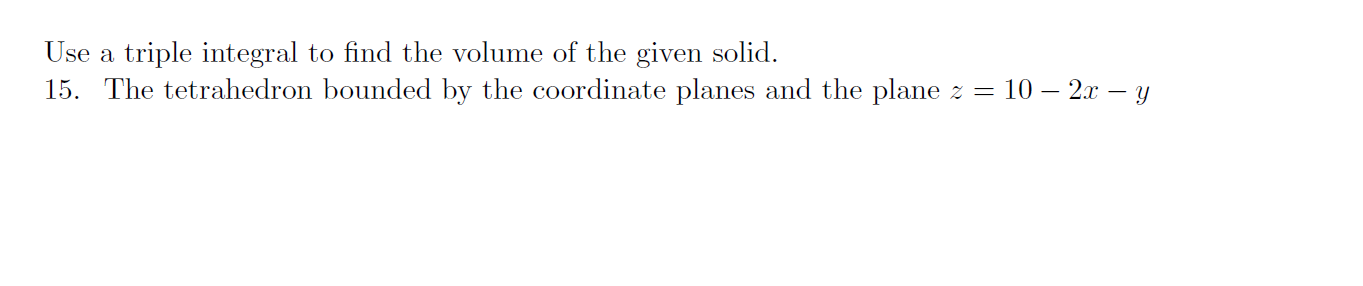### Create an Account

Home / Questions / Use a triple integral to find the volume of the given solid. 15. The tetrahedron bounded b...

# Use a triple integral to find the volume of the given solid. 15. The tetrahedron bounded by the coordinate planes and the plane z = 10 – 2x – y Use a triple integral to find the volume of the given so

Use a triple integral to find the volume of the given solid. 15. The tetrahedron bounded by the coordinate planes and the plane z = 10 – 2x – y
Use a triple integral to find the volume of the given solid. 15. The tetrahedron bounded by the coordinate planes and the plane z = 10 – 2x – yApr 13 2021 View more View LessSubscribe To Get Solution